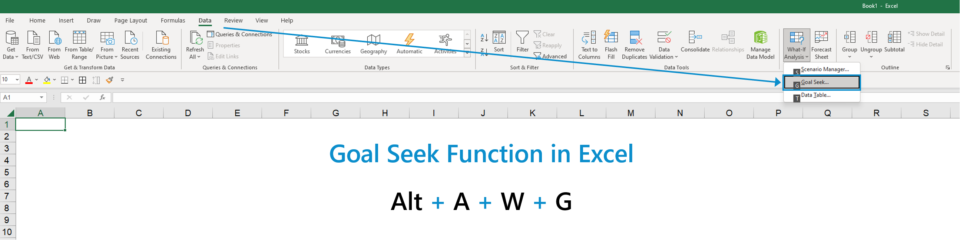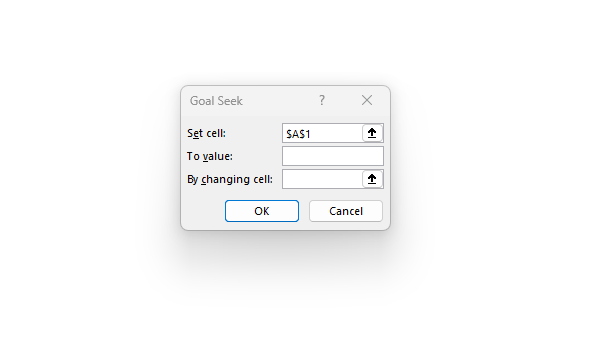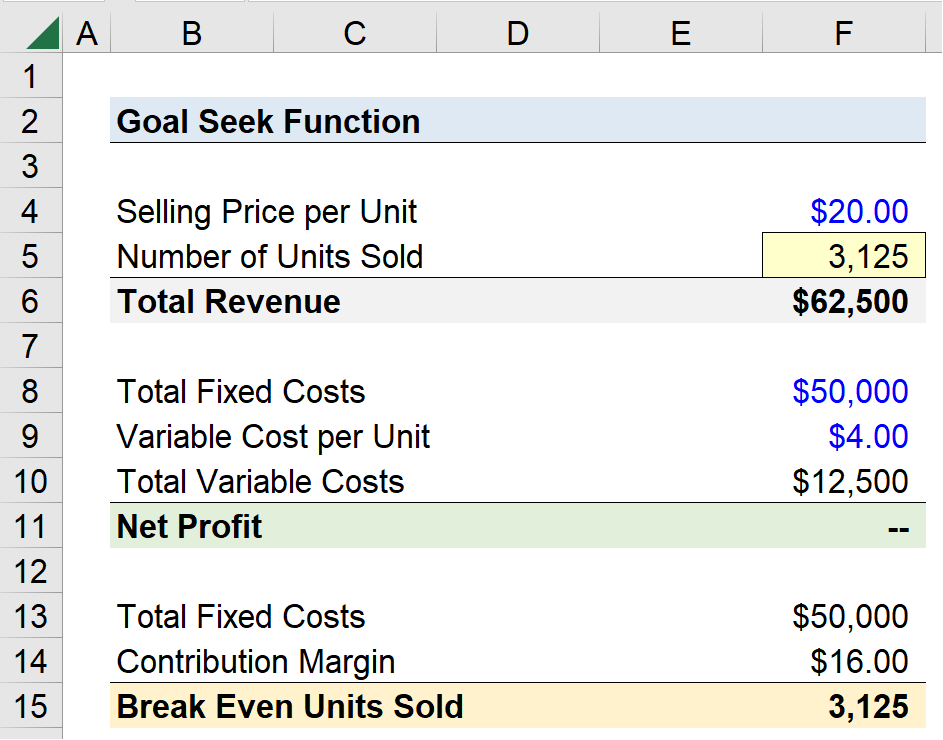Welcome to Wall Street Prep! Use code at checkout for 15% off.# Goal Seek Function

Guide to Understanding the Goal Seek Function in Excel## How to Use Goal Seek Function in Excel (Step-by-Step)

In Excel, the Goal Seek function is a method used to determine the input value that enables the user to reach the desired result.

With Goal Seek, the need for the user to utilize “trial and error” to triangulate the right assumption becomes unnecessary. Further, the Goal Seek function can perform the task far quicker and more accurately.

For example, one use-case of the Goal Seek function is to calculate a company’s break-even units, which is the number of units required to be sold by a company for it to reach a net profit of zero – i.e. the break-even point (BEP).

Using the Goal Seek function can be broken into four steps:

• Step 1: Select the Cell with the Desired Output (i.e. the Goal)
• Step 2: Click on the Output Cell in “Set cell” Selection
• Step 3: Type the Desired Cell Value into the “To value” Box
• Step 4: Enter the Reference for the Variable Cell into the “By changing cell” Box

Once the “Enter” key is then pressed, Excel automatically adjusts the cell value in the variable cell using an iterative approach until the desired output is returned.

## Excel Goal Seek Function: Keyboard Shortcut

To use the Goal Seek function via keyboard shortcuts, start by ensuring the desired output cell is the active selection and then press the following keys:

Goal Seek Function in Excel = Alt ➝ A ➝ W G

Pressing “Alt” displays the ribbon shortcuts in Excel and clicking “A” opens the data tab.

From there, the “W” key opens the “What-If Analysis” menu, in which “Goal Seek…” is the second option to pick from in the list.

Finally, pressing the “G” key selects the Goal Seek function and the following box should open, in which we see the three inputs mentioned earlier.

1. Set cell:” ➝ The cell in which the desired output will be returned.
2. To value:” ➝ The desired value of the cell.
3. “By changing cell:” ➝ The cell that’ll be adjusted in order for the output cell to contain the desired value.## Goal Seek Function Calculator – Excel Model Template

We’ll now move on to a modeling exercise, which you can access by filling out the form below.Submitting...

## Goal Seek Function Excel Tutorial: Break Even Units Sold Example

Suppose an eCommerce company sells its products for \$20.00 each and is attempting to determine the number of units it must sell to break even.

We’ll start by entering the “Selling Price per Unit” into our spreadsheet with a placeholder for the “Number of Units Sold”.

The “Total Revenue” is calculated by multiplying the selling price by the number of units sold.

Our next step is to determine the total costs incurred by the company, which consist of fixed costs and variable costs.

Fixed costs remain constant, irrespective of the unit production, such as the rental expense on a property.

In contrast, variable costs fluctuate based on the production volume. Therefore, an increase in product sales causes more variable costs to be incurred (and vice versa).

The net profit is equal to the total revenue minus the sum of the total fixed costs and the total variable costs.

The total variable costs are equal to the variable cost per unit assumption (\$4.00) multiplied by the number of units sold, which is currently still a placeholder.

In the bottom section, we’ll link our total fixed costs to the section above and calculate our contribution margin – i.e. the selling price per unit less the variable cost per unit – which comes out to be \$16.00.

By dividing the total fixed costs by the contribution margin, we can arrive at the implied break-even units sold, which we’ll use to confirm that our upcoming calculation is correct.

With the net profit cell selected, we’ll open the Goal Seek function box.

1. Set cell:” ➝ Net Profit (F11)
2. To value:” ➝ 0
3. “By changing cell:” ➝ Number of Units Sold (F5)

In closing, we’ve utilized the Goal Seek function in Excel to determine the number of units (3,125 units) required to be sold by our eCommerce company to reach its break-even point.Turbo-charge your time in Excel Used at top investment banks, Wall Street Prep's Excel Crash Course will turn you into an advanced Power User and set you apart from your peers.
Inline Feedbacks